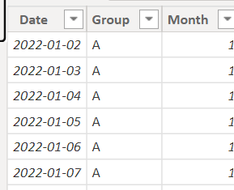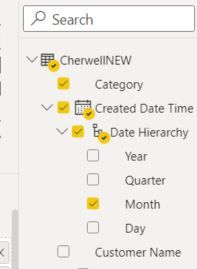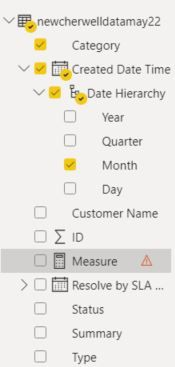cancel
Showing results for
Did you mean:Helper I

## Report Help -

Hi

Im trying to Create a report that lists by month, top 10 categories month by month, some will change each months so in need to reflect this , is this possible?1 ACCEPTED SOLUTIONCommunity Support

Hi  @dommyw277 ,

I created some data:Here are the steps you can follow：

1. Create measure.

``````Count =
var _select=SELECTEDVALUE('Table'[Month])
return
COUNTX(FILTER(ALL('Table'),'Table'[Month]=_select&&'Table'[Group]=MAX('Table'[Group])),[Group])``````
``````rank =
RANKX(ALL('Table'),[Count],,DESC,Dense)``````
``````Flag =
IF(
[rank]<=10,1,0)``````

2. Place [Flag]in Filters, set is=1, apply filter.3. Result:For errors, you can refer to:

https://community.powerbi.com/t5/Desktop/Column-cannot-be-found-or-may-not-be-used-in-this-expressio...

Best Regards,

Liu Yang

If this post helps, then please consider Accept it as the solution to help the other members find it more quickly

11 REPLIES 11Community Support

Hi  @dommyw277 ,

I created some data:Here are the steps you can follow：

1. Create measure.

``````Count =
var _select=SELECTEDVALUE('Table'[Month])
return
COUNTX(FILTER(ALL('Table'),'Table'[Month]=_select&&'Table'[Group]=MAX('Table'[Group])),[Group])``````
``````rank =
RANKX(ALL('Table'),[Count],,DESC,Dense)``````
``````Flag =
IF(
[rank]<=10,1,0)``````

2. Place [Flag]in Filters, set is=1, apply filter.3. Result:For errors, you can refer to:

https://community.powerbi.com/t5/Desktop/Column-cannot-be-found-or-may-not-be-used-in-this-expressio...

Best Regards,

Liu Yang

If this post helps, then please consider Accept it as the solution to help the other members find it more quicklyHelper I

Thank you so much! Works a treatHelper I

So using the table I have called ReportData , the fields are Category and Month , I have this - can anyone help where im going wrong please?

calculate(Sum(ReportData[Category]) ,TOPN(10,all(ReportData[Category]),calculate(Sum(ReportData[Month]) )), values(ReportData[Category]))Super User

Hi,

This measure is correct.  What problem are you facing?

Regards,
Ashish Mathur
http://www.ashishmathur.comHelper I

The issue appears to be that im trying to SUM Text fields so maybe thats why the TOP n argument isnt working?

calculate(Sum(ReportData[Category]) ,TOPN(10,all(ReportData[Category]),calculate(Sum(ReportData[Month]) )), values(ReportData[Category]))Super User

Hi,

Does this work?

=calculate(countrows(ReportData) ,TOPN(10,all(ReportData[Category]),calculate(Sum(ReportData[Month]) )), values(ReportData[Category]))

Regards,
Ashish Mathur
http://www.ashishmathur.comHelper I

Hi, so ReportData is replaced by NewCherwell however i get these messages. Maybe because Month is a part of created date time?Super User

Hi,

Regards,
Ashish Mathur
http://www.ashishmathur.comHelper I

Hi im getting this message:

ReportData is replaced by CherwellNEW for infoHelper IHi, what do i put where the value is?Super User

@dommyw277 , Create a TOPN measure and display is with month and category

calculate(Sum(Table[Value]) ,TOPN(1,all(Table[category]),calculate(Sum(Table[Value]) )), values(Table[category]))

or refer this

https://www.sqlbi.com/articles/filtering-the-top-3-products-for-each-category-in-power-bi/

!! Microsoft Fabric !!
Microsoft Power BI Learning Resources, 2023 !!
Learn Power BI - Full Course with Dec-2022, with Window, Index, Offset, 100+ Topics !!
Did I answer your question? Mark my post as a solution! Appreciate your Kudos !! Proud to be a Super User! !!